Introduction to Circuit Analysis
Getting Started

 Outline: What is a circuit? The purpose of building a circuit What are elements? The Definition of Current Voltage Energy and Power

What is a Circuit?
```
A circuit is a colletion of of electrical elements interconnected in some way,
where at least one closed path exists so that current may flow.

This IS a circuit: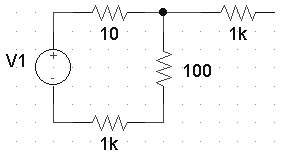This is NOT a circuit: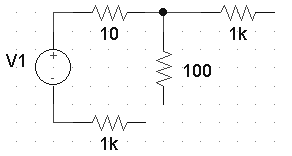```
Top of Page

What is the purpose of building a circuit?
```
The purpose of contructing circuits is to direct current in a specific way such that
work can be performed.

This is similar to damming a river and forcing the water to flow
through turbines which are in turn connected to generators.

The idea is that we want to take advantage of our sources of energy
to perform some useful work.  In this course, our sources of energy
are batteries and power supplies.

Useful work is getting done because current
is flowing from V1 to the resisitor (resistor may
be a lightbulb or a stove burner):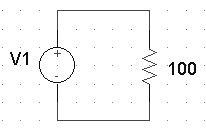Current is just flowing into the Ground,
i.e. nothing useful is happening: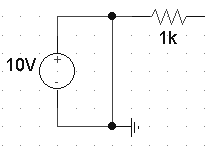```
Top of Page

What are elements?
```
Elements are the individual components or building blocks of a circuit.

Some Circuit Components:

Resistor: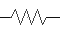capacitor: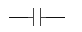Inductor: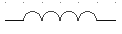Battery: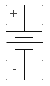Generator: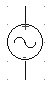Diode: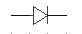Inverter: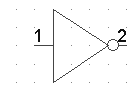Transistor: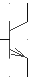```
Top of Page

Definition of Current
```
Current is the flow of charge.  It can be defined as either the flow
of negative charge (the flow of electrons) or it can be defined as the
flow of positive charge.

The military typically defines current as the flow of negative charge, however
most academic institutions define current as the flow of positive charge.  Both
conventions are valid when doing circuit analysis.
```
In this class we will ALWAYS assume current is the flow of POSITIVE charge.

Positive charge going left to right is exactly the same as
negative charge going from right to left:A Coulomb is the unit used to measure charge.

1 Electron = 1.6021 X 10-19 Coulombs
1 Coulomb = 6.24 X 1018 Electrons
```In this class we will describe charge in terms of Coulombs.

The Symbol for charge is Q

For example:  Q = 4C   <--- This means the amount of charge is 4 Coulombs

In this class we are interested in the FLOW OF CHARGE.  Static charge (charge that
isn't moving) isn't very useful for getting work done.

Charge in motion is called Current.```

Current is the flow of charge, i.e. it is the rate of charge moving:

i is the symbol for current:

i = dQ/dt

The unit of Current is the Ampere (or Amp for short):
An Ampere = 1Coulomb/second

1 Amp = 1 C/sec.
5 Amps = 5 C/sec.

The following two currents are exactly the same: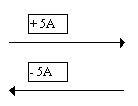Top of Page

Definition of Voltage

What moves water from one point to another?

The force of Gravity forces water to move

from a higher potential energy to a lower

potential energy.

What moves electrical charge from one point to another?

EMF (Electromotive Force) is the

force which moves charge from a

higher electrical potential energy

to a lower potential energy.

Recall that Work = (Force * Distance)

When an EMF is present and current moves through an element (such as a resistor) then we say that work is being done.

So What is Voltage?

Voltage is the amount of work required
to move 1 Coulomb of charge from one point to another.

Volt = joule/coulomb
Voltage is defined across an element. It is measured between two points.

Consider the picture below: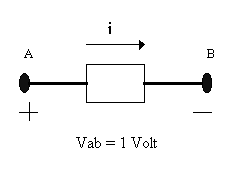There is a one volt difference between point-A and point-B
Therefore to move one coulumb of charge from A to B requires 1 joule.

Voltage is always defined between two points, in other words, you need a relative point.
In the picture above Vab = 10 V
(Vab means the voltage at pt-A relative to pt-B)
Or we can say the voltage at pt-A is 10 volts above the voltage at pt-B

Similarly in the picture above Vba = -10 V
(Vba means the voltage at pt-B relative to pt-A)
Or we can say the voltage at pt-B is 10 volts below the voltage at pt-A

Vab = -Vba

The following two circuits are equivalent: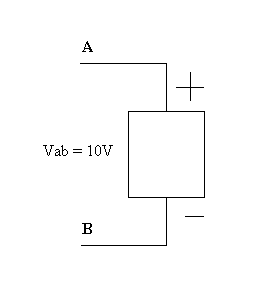The following two circuits are also equivalent: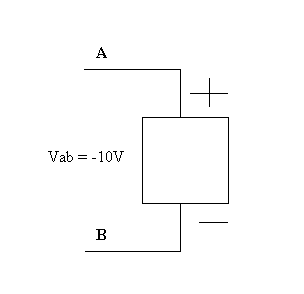Top of Page

Calculating Energy and Power

A circuit element eithers absorbs or delivers energy:

 Here the element is absorbing EnergyCurrent flows into the positive terminal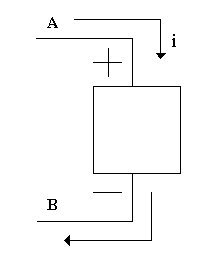Here the element is delivering EnergyCurrent flows into the negative terminal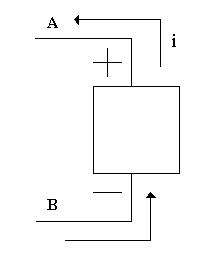Definition of Power:
The rate that energy is absorbed or delivered by an element.

Let's derive an equation for Power:

V = J/C
dWork = Vdq
dWork/dt = V dq/dt
dWork/dt is Power and dq/dt is current, therefore:

Power = VI

The following table shows some examples of how to calculate power:

 Here the element is absorbing EnergyPabs = VI = 5V(4A)= 20 Watts, or we can say Pdel = -20 Watts Note: Pabsorbed = -Pdelivered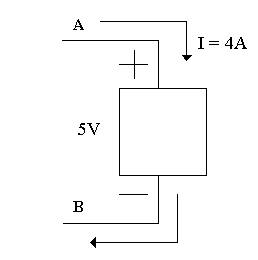Here the element is delivering EnergyPdel = VI = 8V(2A)= 16 Watts, or we can say Pabs = -16 Watts Note: Pabsorbed = -Pdelivered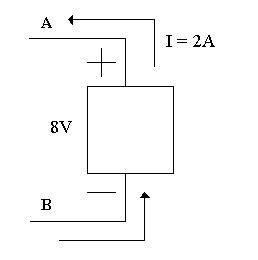Here the element is absorbing EnergyPabs = VI = -6V(-4A)= 24 Watts, or we can say Pdel = -24 Watts Note: Pabsorbed = -Pdelivered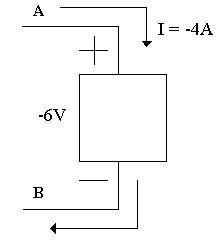Here the element is delivering EnergyPdel = VI = -9V(5A)= -45 Watts, or we can say Pabs = 45 Watts Note: Pabsorbed = -Pdelivered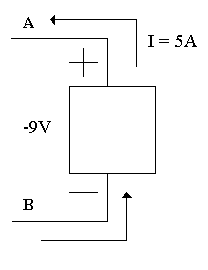Top of Page

## Study Problems

After clicking on the following link enter 1-8 for the problem and 1 for the step:
Study Problem 1-8
After clicking on the following link enter 1-9 for the problem and 1 for the step:
Study Problem 1-9

Back To Index